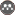Volume 18, Issue 68 (summer 2014)                   JWSS 2014, 18(68): 121-131 | Back to browse issues page

BibTeX | RIS | EndNote | Medlars | ProCite | Reference Manager | RefWorks
Send citation to:Abdi Dehkordi M, Dehghani A A, Meftah M, Kahe M, Hesam M, Dehghani N. Use of Fuzzy Clustering Algorithm for Estimating the Daily Suspended Load (Case Study: Kasilain Basin). JWSS. 2014; 18 (68) :121-131
URL: http://jstnar.iut.ac.ir/article-1-2782-en.html
Abstract:   (9249 Views)
In many water resource projects such as dams, flood control, navigability, river aesthetics, environmental issues and the estimation of suspended load have great importance. The complexity of sediment behavior and mathematical and physical model inability in simulation of sedimentation processes have led to the development of new technologies such as fuzzy logic which has the ability to identify nonlinear relationship between input and output variables. In this study, the application of fuzzy clustering algorithm in estimating the annual amount of sediment was studied. So, the corresponding data of flow and sediment discharge of Valykben station in kasilian basin during 1349-1350 till 1353-1354 period was daily determined. The data was divided in two groups i. e. 75% as training data and 25% for test data. Then, the efficiency of model was obtained by using statistical parameters such as correlation coefficient, nash-satklyf coefficient, mean square error root and variance ratio. The result showed that the classification of data on the annual time scale and use of fuzzy clustering algorithm can estimate 0.49 values of the measured annually suspended sediment transport. Furthermore, on the same scale of classification, i.e. annual scale, this value was obtained 0.19. Thus, using fuzzy clustering algorithm can lead to higher accuracy and reliability than rating curve method, which is suggested for estimating suspended sediment transport.